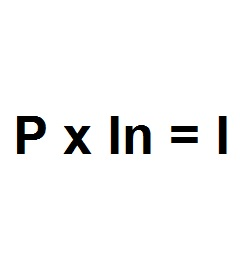Formula - Simple Interest | Propertylogy
Don't Miss

# Simple Interest

By on August 18, 2018P x In = I

Where:

P = Principal

In = Interest rate

I = Interest

Simple interest is the most basic interest rate structure which takes the loan principal multiplied by the interest rate, to obtain the interest cost.

This means that \$100 x 5% will equal to \$5.

Simple interest is the interest rate that everyone intuitively understands and often assume it’s what lenders are charging borrowers at.

It’s a huge irony that very few (or none) of the loans available from the big banks are structured with simple interest rates.

Even when a loan officer presents a loan to a borrower with simple interest, it is more likely that it is not. And rather converted to simple interest so that a borrower would know how much interest is being charged.

So be mindful of that when presented with loans, especially mortgages, with simple interest.

You May Also Like...##### How to burn more calories in the office

Send this to a friend